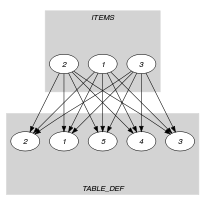## 5.148. elements_sparse

Origin
Constraint

$\mathrm{𝚎𝚕𝚎𝚖𝚎𝚗𝚝𝚜}_\mathrm{𝚜𝚙𝚊𝚛𝚜𝚎}\left(\mathrm{𝙸𝚃𝙴𝙼𝚂},\mathrm{𝚃𝙰𝙱𝙻𝙴},\mathrm{𝙳𝙴𝙵𝙰𝚄𝙻𝚃}\right)$

Arguments
 $\mathrm{𝙸𝚃𝙴𝙼𝚂}$ $\mathrm{𝚌𝚘𝚕𝚕𝚎𝚌𝚝𝚒𝚘𝚗}\left(\mathrm{𝚒𝚗𝚍𝚎𝚡}-\mathrm{𝚍𝚟𝚊𝚛},\mathrm{𝚟𝚊𝚕𝚞𝚎}-\mathrm{𝚍𝚟𝚊𝚛}\right)$ $\mathrm{𝚃𝙰𝙱𝙻𝙴}$ $\mathrm{𝚌𝚘𝚕𝚕𝚎𝚌𝚝𝚒𝚘𝚗}\left(\mathrm{𝚒𝚗𝚍𝚎𝚡}-\mathrm{𝚒𝚗𝚝},\mathrm{𝚟𝚊𝚕𝚞𝚎}-\mathrm{𝚒𝚗𝚝}\right)$ $\mathrm{𝙳𝙴𝙵𝙰𝚄𝙻𝚃}$ $\mathrm{𝚒𝚗𝚝}$
Restrictions
 $\mathrm{𝚛𝚎𝚚𝚞𝚒𝚛𝚎𝚍}$$\left(\mathrm{𝙸𝚃𝙴𝙼𝚂},\left[\mathrm{𝚒𝚗𝚍𝚎𝚡},\mathrm{𝚟𝚊𝚕𝚞𝚎}\right]\right)$ $\mathrm{𝙸𝚃𝙴𝙼𝚂}.\mathrm{𝚒𝚗𝚍𝚎𝚡}\ge 1$ $\mathrm{𝚛𝚎𝚚𝚞𝚒𝚛𝚎𝚍}$$\left(\mathrm{𝚃𝙰𝙱𝙻𝙴},\left[\mathrm{𝚒𝚗𝚍𝚎𝚡},\mathrm{𝚟𝚊𝚕𝚞𝚎}\right]\right)$ $\mathrm{𝚃𝙰𝙱𝙻𝙴}.\mathrm{𝚒𝚗𝚍𝚎𝚡}\ge 1$ $\mathrm{𝚍𝚒𝚜𝚝𝚒𝚗𝚌𝚝}$$\left(\mathrm{𝚃𝙰𝙱𝙻𝙴},\mathrm{𝚒𝚗𝚍𝚎𝚡}\right)$
Purpose

All the items of $\mathrm{𝙸𝚃𝙴𝙼𝚂}$ should be equal to one of the entries of the table $\mathrm{𝚃𝙰𝙱𝙻𝙴}$ or to the default value $\mathrm{𝙳𝙴𝙵𝙰𝚄𝙻𝚃}$ if the entry $\mathrm{𝙸𝚃𝙴𝙼𝚂}.\mathrm{𝚒𝚗𝚍𝚎𝚡}$ does not occurs among the values of the index attribute of the $\mathrm{𝚃𝙰𝙱𝙻𝙴}$ collection.

Example
$\left(\begin{array}{c}〈\begin{array}{cc}\mathrm{𝚒𝚗𝚍𝚎𝚡}-8\hfill & \mathrm{𝚟𝚊𝚕𝚞𝚎}-9,\hfill \\ \mathrm{𝚒𝚗𝚍𝚎𝚡}-3\hfill & \mathrm{𝚟𝚊𝚕𝚞𝚎}-5,\hfill \\ \mathrm{𝚒𝚗𝚍𝚎𝚡}-2\hfill & \mathrm{𝚟𝚊𝚕𝚞𝚎}-5\hfill \end{array}〉,\hfill \\ 〈\begin{array}{cc}\mathrm{𝚒𝚗𝚍𝚎𝚡}-1\hfill & \mathrm{𝚟𝚊𝚕𝚞𝚎}-6,\hfill \\ \mathrm{𝚒𝚗𝚍𝚎𝚡}-2\hfill & \mathrm{𝚟𝚊𝚕𝚞𝚎}-5,\hfill \\ \mathrm{𝚒𝚗𝚍𝚎𝚡}-4\hfill & \mathrm{𝚟𝚊𝚕𝚞𝚎}-2,\hfill \\ \mathrm{𝚒𝚗𝚍𝚎𝚡}-8\hfill & \mathrm{𝚟𝚊𝚕𝚞𝚎}-9\hfill \end{array}〉,5\hfill \end{array}\right)$

The $\mathrm{𝚎𝚕𝚎𝚖𝚎𝚗𝚝𝚜}_\mathrm{𝚜𝚙𝚊𝚛𝚜𝚎}$ constraint holds since:

• The first and third items (items $〈\mathrm{𝚒𝚗𝚍𝚎𝚡}-8\mathrm{𝚟𝚊𝚕𝚞𝚎}-9〉$ and $〈\mathrm{𝚒𝚗𝚍𝚎𝚡}-2\mathrm{𝚟𝚊𝚕𝚞𝚎}-5〉$) of its $\mathrm{𝙸𝚃𝙴𝙼𝚂}$ collection respectively correspond to the fourth and second item of its $\mathrm{𝚃𝙰𝙱𝙻𝙴}$ collection.

• The $\mathrm{𝚒𝚗𝚍𝚎𝚡}$ attribute of the second item of its $\mathrm{𝙸𝚃𝙴𝙼𝚂}$ collection (i.e., value 3) does not correspond to any index of the $\mathrm{𝚃𝙰𝙱𝙻𝙴}$ collection. Therefore the $\mathrm{𝚟𝚊𝚕𝚞𝚎}$ attribute of the second item of the $\mathrm{𝙸𝚃𝙴𝙼𝚂}$ collection is set the the default value 5 given by the last argument of the $\mathrm{𝚎𝚕𝚎𝚖𝚎𝚗𝚝𝚜}_\mathrm{𝚜𝚙𝚊𝚛𝚜𝚎}$ constraint.

Typical
 $|\mathrm{𝙸𝚃𝙴𝙼𝚂}|>1$ $\mathrm{𝚛𝚊𝚗𝚐𝚎}$$\left(\mathrm{𝙸𝚃𝙴𝙼𝚂}.\mathrm{𝚟𝚊𝚕𝚞𝚎}\right)>1$ $|\mathrm{𝚃𝙰𝙱𝙻𝙴}|>1$ $\mathrm{𝚛𝚊𝚗𝚐𝚎}$$\left(\mathrm{𝚃𝙰𝙱𝙻𝙴}.\mathrm{𝚟𝚊𝚕𝚞𝚎}\right)>1$
Symmetries
• Items of $\mathrm{𝙸𝚃𝙴𝙼𝚂}$ are permutable.

• Items of $\mathrm{𝚃𝙰𝙱𝙻𝙴}$ are permutable.

• All occurrences of two distinct values in $\mathrm{𝙸𝚃𝙴𝙼𝚂}.\mathrm{𝚟𝚊𝚕𝚞𝚎}$, $\mathrm{𝚃𝙰𝙱𝙻𝙴}.\mathrm{𝚟𝚊𝚕𝚞𝚎}$ or $\mathrm{𝙳𝙴𝙵𝙰𝚄𝙻𝚃}$ can be swapped; all occurrences of a value in $\mathrm{𝙸𝚃𝙴𝙼𝚂}.\mathrm{𝚟𝚊𝚕𝚞𝚎}$, $\mathrm{𝚃𝙰𝙱𝙻𝙴}.\mathrm{𝚟𝚊𝚕𝚞𝚎}$ or $\mathrm{𝙳𝙴𝙵𝙰𝚄𝙻𝚃}$ can be renamed to any unused value.

Usage

Used for replacing several $\mathrm{𝚎𝚕𝚎𝚖𝚎𝚗𝚝}$ constraints sharing exactly the same sparse table by a single constraint.

Reformulation

Let ${𝙸}_{k}$ and ${𝚅}_{k}$ respectively denote $\mathrm{𝙸𝚃𝙴𝙼𝚂}\left[k\right].\mathrm{𝚒𝚗𝚍𝚎𝚡}$ and $\mathrm{𝙸𝚃𝙴𝙼𝚂}\left[k\right].\mathrm{𝚟𝚊𝚕𝚞𝚎}$ $\left(k\in \left[1,|\mathrm{𝙸𝚃𝙴𝙼𝚂}|\left[\right]\right)$. The $\mathrm{𝚎𝚕𝚎𝚖𝚎𝚗𝚝𝚜}_\mathrm{𝚜𝚙𝚊𝚛𝚜𝚎}$$\left(\mathrm{𝙸𝚃𝙴𝙼𝚂},\mathrm{𝚃𝙰𝙱𝙻𝙴},\mathrm{𝙳𝙴𝙵𝙰𝚄𝙻𝚃}\right)$ constraint can be expressed in term of $|\mathrm{𝙸𝚃𝙴𝙼𝚂}|\left[$ reified constraints of the form:

$\left(\left({𝙸}_{k}=\mathrm{𝚃𝙰𝙱𝙻𝙴}\left[1\right].\mathrm{𝚒𝚗𝚍𝚎𝚡}\wedge {𝚅}_{k}=\mathrm{𝚃𝙰𝙱𝙻𝙴}\left[1\right].\mathrm{𝚟𝚊𝚕𝚞𝚎}\right)\vee$

$\left({𝙸}_{k}=\mathrm{𝚃𝙰𝙱𝙻𝙴}\left[2\right].\mathrm{𝚒𝚗𝚍𝚎𝚡}\wedge {𝚅}_{k}=\mathrm{𝚃𝙰𝙱𝙻𝙴}\left[2\right].\mathrm{𝚟𝚊𝚕𝚞𝚎}\right)\vee$

$\cdots$

$\left({𝙸}_{k}=\mathrm{𝚃𝙰𝙱𝙻𝙴}\left[|\mathrm{𝚃𝙰𝙱𝙻𝙴}|\right].\mathrm{𝚒𝚗𝚍𝚎𝚡}\wedge {𝚅}_{k}=\mathrm{𝚃𝙰𝙱𝙻𝙴}\left[\mathrm{𝚃𝙰𝙱𝙻𝙴}|\right].\mathrm{𝚟𝚊𝚕𝚞𝚎}\right)\right)\vee$

$\left(\left({𝙸}_{k}\ne \mathrm{𝚃𝙰𝙱𝙻𝙴}\left[1\right].\mathrm{𝚒𝚗𝚍𝚎𝚡}\right)\wedge$

$\left({𝙸}_{k}\ne \mathrm{𝚃𝙰𝙱𝙻𝙴}\left[2\right].\mathrm{𝚒𝚗𝚍𝚎𝚡}\right)\wedge$

$\cdots$

$\left({𝙸}_{k}\ne \mathrm{𝚃𝙰𝙱𝙻𝙴}\left[|\mathrm{𝚃𝙰𝙱𝙻𝙴}|\right].\mathrm{𝚒𝚗𝚍𝚎𝚡}\right)\wedge$

$\left({𝚅}_{k}=\mathrm{𝙳𝙴𝙵𝙰𝚄𝙻𝚃}\right)\right)$.

Keywords
Derived Collections
 $\mathrm{𝚌𝚘𝚕}\left(\begin{array}{c}\mathrm{𝙳𝙴𝙵}-\mathrm{𝚌𝚘𝚕𝚕𝚎𝚌𝚝𝚒𝚘𝚗}\left(\mathrm{𝚒𝚗𝚍𝚎𝚡}-\mathrm{𝚒𝚗𝚝},\mathrm{𝚟𝚊𝚕𝚞𝚎}-\mathrm{𝚒𝚗𝚝}\right),\hfill \\ \mathrm{𝚒𝚝𝚎𝚖}\left(\mathrm{𝚒𝚗𝚍𝚎𝚡}-0,\mathrm{𝚟𝚊𝚕𝚞𝚎}-\mathrm{𝙳𝙴𝙵𝙰𝚄𝙻𝚃}\right)\right]\hfill \end{array}\right)$ $\mathrm{𝚌𝚘𝚕}\left(\begin{array}{c}\mathrm{𝚃𝙰𝙱𝙻𝙴}_\mathrm{𝙳𝙴𝙵}-\mathrm{𝚌𝚘𝚕𝚕𝚎𝚌𝚝𝚒𝚘𝚗}\left(\mathrm{𝚒𝚗𝚍𝚎𝚡}-\mathrm{𝚍𝚟𝚊𝚛},\mathrm{𝚟𝚊𝚕𝚞𝚎}-\mathrm{𝚍𝚟𝚊𝚛}\right),\hfill \\ \left[\begin{array}{c}\mathrm{𝚒𝚝𝚎𝚖}\left(\mathrm{𝚒𝚗𝚍𝚎𝚡}-\mathrm{𝚃𝙰𝙱𝙻𝙴}.\mathrm{𝚒𝚗𝚍𝚎𝚡},\mathrm{𝚟𝚊𝚕𝚞𝚎}-\mathrm{𝚃𝙰𝙱𝙻𝙴}.\mathrm{𝚒𝚗𝚍𝚎𝚡}\right),\hfill \\ \mathrm{𝚒𝚝𝚎𝚖}\left(\mathrm{𝚒𝚗𝚍𝚎𝚡}-\mathrm{𝙳𝙴𝙵}.\mathrm{𝚒𝚗𝚍𝚎𝚡},\mathrm{𝚟𝚊𝚕𝚞𝚎}-\mathrm{𝙳𝙴𝙵}.\mathrm{𝚟𝚊𝚕𝚞𝚎}\right)\hfill \end{array}\right]\hfill \end{array}\right)$
Arc input(s)

$\mathrm{𝙸𝚃𝙴𝙼𝚂}$ $\mathrm{𝚃𝙰𝙱𝙻𝙴}_\mathrm{𝙳𝙴𝙵}$

Arc generator
$\mathrm{𝑃𝑅𝑂𝐷𝑈𝐶𝑇}$$↦\mathrm{𝚌𝚘𝚕𝚕𝚎𝚌𝚝𝚒𝚘𝚗}\left(\mathrm{𝚒𝚝𝚎𝚖𝚜},\mathrm{𝚝𝚊𝚋𝚕𝚎}_\mathrm{𝚍𝚎𝚏}\right)$

Arc arity
Arc constraint(s)
 $•\mathrm{𝚒𝚝𝚎𝚖𝚜}.\mathrm{𝚟𝚊𝚕𝚞𝚎}=\mathrm{𝚝𝚊𝚋𝚕𝚎}_\mathrm{𝚍𝚎𝚏}.\mathrm{𝚟𝚊𝚕𝚞𝚎}$ $•\mathrm{𝚒𝚝𝚎𝚖𝚜}.\mathrm{𝚒𝚗𝚍𝚎𝚡}=\mathrm{𝚝𝚊𝚋𝚕𝚎}_\mathrm{𝚍𝚎𝚏}.\mathrm{𝚒𝚗𝚍𝚎𝚡}\vee \mathrm{𝚝𝚊𝚋𝚕𝚎}_\mathrm{𝚍𝚎𝚏}.\mathrm{𝚒𝚗𝚍𝚎𝚡}=0$
Graph property(ies)
$\mathrm{𝐍𝐒𝐎𝐔𝐑𝐂𝐄}$$=|\mathrm{𝙸𝚃𝙴𝙼𝚂}|$

Graph model

An item of the $\mathrm{𝙸𝚃𝙴𝙼𝚂}$ collection may have up to two successors (see for instance the third item of the $\mathrm{𝙸𝚃𝙴𝙼𝚂}$ collection of the Example slot). Therefore we use the graph property $\mathrm{𝐍𝐒𝐎𝐔𝐑𝐂𝐄}=|\mathrm{𝙸𝚃𝙴𝙼𝚂}|$ for enforcing the fact that each item of the $\mathrm{𝙸𝚃𝙴𝙼𝚂}$ collection has at least one successor.

Parts (A) and (B) of Figure 5.148.1 respectively show the initial and final graph associated with the Example slot. Since we use the $\mathrm{𝐍𝐒𝐎𝐔𝐑𝐂𝐄}$ graph property, the vertices of the final graph are drawn with a double circle.

##### Figure 5.148.1. Initial and final graph of the $\mathrm{𝚎𝚕𝚎𝚖𝚎𝚗𝚝𝚜}_\mathrm{𝚜𝚙𝚊𝚛𝚜𝚎}$ constraint(a) (b)
Signature

On the one hand note that $\mathrm{𝙸𝚃𝙴𝙼𝚂}$ is equal to the number of sources of the initial graph. On the other hand note that, in the initial graph, all the vertices that are not sources correspond to sinks. Since isolated vertices are eliminated from the final graph the sinks of the initial graph cannot become sources of the final graph. Therefore the maximum number of sources of the final graph is equal to $\mathrm{𝙸𝚃𝙴𝙼𝚂}$. We can rewrite $\mathrm{𝐍𝐒𝐎𝐔𝐑𝐂𝐄}=|\mathrm{𝙸𝚃𝙴𝙼𝚂}|$ to $\mathrm{𝐍𝐒𝐎𝐔𝐑𝐂𝐄}\ge |\mathrm{𝙸𝚃𝙴𝙼𝚂}|$ and simplify $\underline{\overline{\mathrm{𝐍𝐒𝐎𝐔𝐑𝐂𝐄}}}$ to $\overline{\mathrm{𝐍𝐒𝐎𝐔𝐑𝐂𝐄}}$.